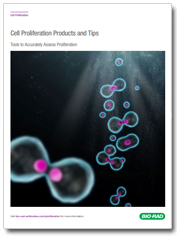# Protocol: Determining Optimum Length of Incubation, Plating Density

s

### Cell Proliferation Products and TipsTools to Accurately Assess Proliferation

## Overview

The two variables which most affect the response of cells to alamarBlue are length of incubation time and number of cells plated. It is recommended that the plating density and incubation time be determined for each cell line using the following procedure:

1. Harvest cells which are in log phase growth stage and determine cell count. Plate cells at various densities, above and below the cell density expected to be used.
2. Aseptically add alamarBlue in an amount equal to 10% of the volume in the well.
3. Return plates to incubator. Remove the plate and measure fluorescence/absorbance each hour following plating for the first 6-8 hr. It is also recommended that the plates remain in incubation overnight and measurements be made the following day at 24 hr. Two kinds of information can be obtained from this data:

(i) for any given incubation time selected, the range in cell density relating cell number to alamarBlue reduction can be determined by a linear response.

(ii) for any given cell density selected, the incubation time can be determined as the time taken for the control cells to turn the indicator from the oxidized (blue) form to the fully reduced (red) form.

1. Measure absorbance at a wavelength of 570 nm and 600 nm; or measure fluorescence with an excitation wavelength at 530-560 nm and emission wavelength at 590 nm.
2. Calculate the percentage reduction of alamarBlue at each cell density or incubation period.

a. Equation 1 calculates percentage reduction of alamarBlue using absorbance

Percentage reduction of alamarBlue (equation 1)

=

(O2 x A1) - (O1 x A2)

(R1 x N2) - (R2 x N1)

x100

Where:

• O1 = molar extinction coefficient (E) of oxidized alamarBlue (blue) at 570 nm*
• O2 = E of oxidized alamarBlue at 600 nm*
• R1 = E of reduced alamarBlue (red) at 570 nm
• R2 = E of reduced alamarBlue at 600 nm
• A1 = absorbance of test wells at 570 nm
• A2 = absorbance of test wells at 600 nm
• N1 = absorbance of negative control well (media plus alamarBlue but no cells) at 570 nm
• N2 = absorbance of negative control well (media plus alamarBlue but no cells) at 600 nm

* Only one appropriate substitute wavelength may be used.

### Example data:

•  O1 = 570 nm O2 = 600 nm
•  O1 = 80586 (see table in general method for oxidation coefficients)
•  O2 = 117216
•  R1 = 155677
•  R2 = 14652
•  A1 = 0.61 observed absorbance reading for test well
•  A2 = 0.42 observed absorbance reading for test well
•  N1 = 0.44 observed absorbance reading for negative control well
•  N2 = 0.64 observed absorbance reading for negative control well

To calculate percent reduced using equation 1:

 Percentage reduction  = (117,216 x 0.61) - (80,586 x 0.42) (155,677 x 0.64) - (14,652 x 0.44) x 100 = 71,502 - 33,846  99,633 - 6.446 x 100 = 0.404 x 100 = 40%

Absorbance 560 nm Absorbance 605 nm
10% alamarBlue in 100 µl media 0.474 0.815
100 µl media 0.082 0.037
Test well after 60 min incubation 0.639 0.889
Test well after 120 min incubation 0.797 0.893

Molar extinction coefficients for alamarBlue at different wavelengths

b) To calculate percentage reduction of alamarBlue using fluorescence, use equation 2:

Percentage reduction of alamarBlue (equation 2)

=

FI 590 of test agent - FI 590 untreated control

FI 590 of 100% reduced alamarBlue - FI 590 untreated control

x100

Where: FI 590 = Fluorescent Intensity at 590 nm emission (560 nm excitation).

### Example data:

• Media RPMI 1640. One sample autoclaved for 15 min to produce reduced form (100% reduced alamarBlue).
• Fluorescence 100% reduced alamarBlue = 58796
• Fluorescence of uninoculated broth (untreated control) = 6472
• Fluorescence of treated sample = 25670
 Percentage reduction of alamarBlue = 25670 - 6472 58796 - 6472 x 100 = 36.7%

1. Plot a graph of percentage reduction of alamarBlue at each cell density or incubation period.

a) For experiments to determine optimum cell density, plot log of cell density on the x axis, and percentage reduction on the y axis.

b) For experiments to determine the optimum incubation time, plot the number of hours on the x axis, and the percentage reduction on the y axis.

c) Use the graphs to determine optimum cell density or incubation period.

Related products